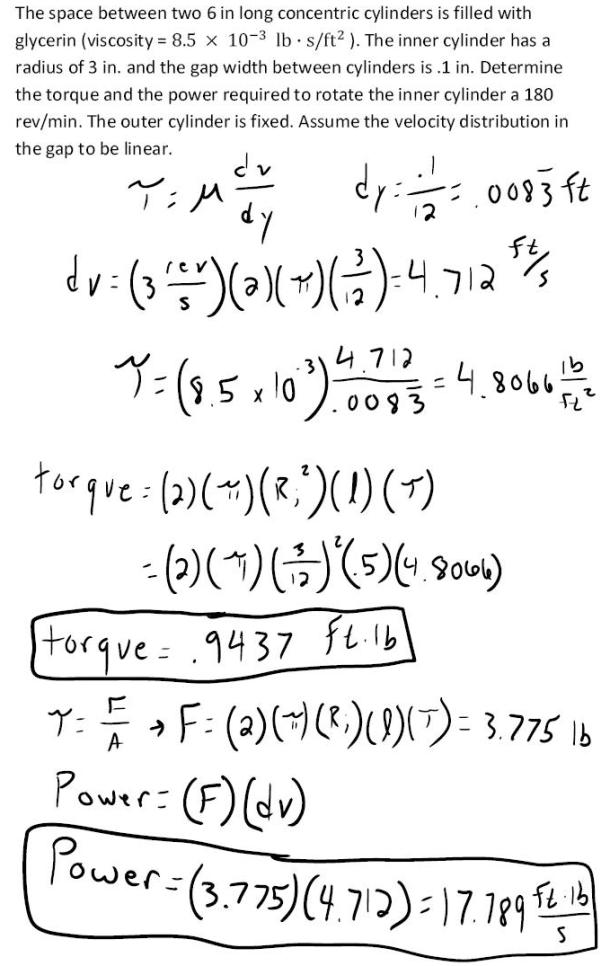fluid mechanics math problems engineering equations fluid mechanics basic mechanics school homework engineering math fluids formulas fluid mechanics problems fluid mechanics problem solutions to fluids problems full solution fluid mechanics engineering fluids problem solution fluid mechanics math problems engineering equations fluid mechanics basic mechanics school homework engineering math fluid mechanics formulas fluid problems fluid mechanics problem solutions to fluid mechanics problems full solution fluid mechanics engineering fluid mechanics problem solution
fluid mechanics math problems engineering equations fluid mechanics basic mechanics school homework engineering math fluids formulas fluid mechanics problems fluid mechanics problem solutions to fluids problems full solution fluid mechanics engineering fluids problem solution fluid mechanics math problems engineering equations fluid mechanics basic mechanics school homework engineering math fluid mechanics formulas fluid problems fluid mechanics problem solutions to fluid mechanics problems full solution fluid mechanics engineering fluid mechanics problem solution
Highalphabet Home Page fluid mechanics solutions fluids math solved Fluid Mechanics Page 1
The space between two 6 in long concentric cylinders is filled with glycerin (viscosity= 8.5x10^-3 lb s/ft^2). The inner cylinder has a radius of 3 in. and the gap width between cylinders in .1 in. Determine the torque and the power required to rotate the inner cylinder at 180 rev/min. The outer cylinder is fixed. Assume the velocity distribution in the gap to be linear.The space between two 6 in long concentric cylinders is filled with glycerin (viscosity= 8.5x10^-3 lb s/ft^2). The inner cylinder has a radius of 3 in. and the gap width between cylinders in .1 in. Determine the torque and the power required to rotate the inner cylinder at 180 rev/min. The outer cylinder is fixed. Assume the velocity distribution in the gap to be linear.